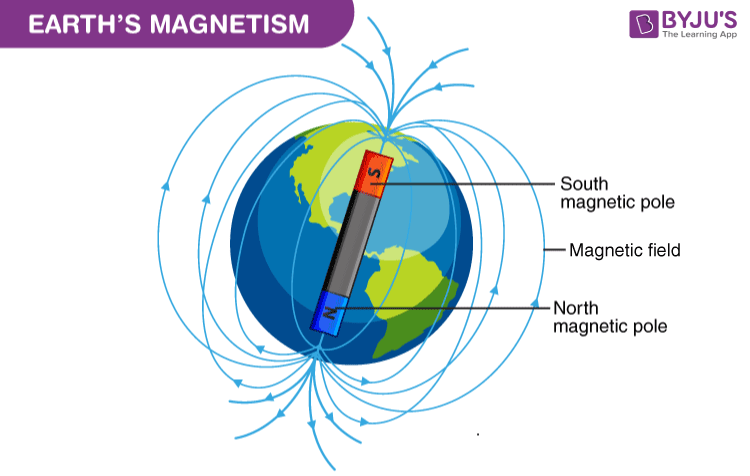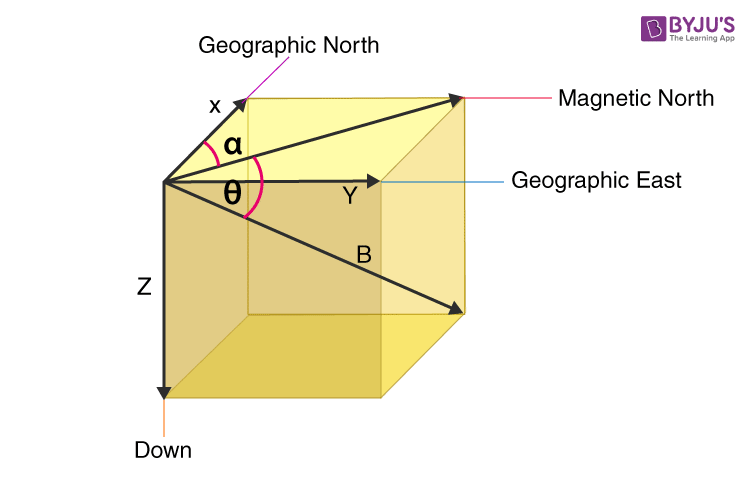# Magnetic Field of Earth - Earth's Magnetism

If you have ever used a compass (either the traditional mechanical one or the one built in your Smartphone), then you know it always points north. If you suspend a refrigerator magnet, it will also point north. This suggests that there is a magnetic field all around us generated by the earth under your feet!. As we know the presence of a magnetic field acts like a protective shield around the Earth, this earth’s magnetic field repels and traps charged particles from the Sun. In this article, let us know in detail about the earth’s magnetic field.

## What Is Earth’s Magnetic Field

Earth’s magnetic field is also referred to as the geomagnetic field. The earth’s magnetic field extends millions of kilometers into outer space and looks very much like a bar magnet. The earth’s south magnetic pole is actually near the North Pole and the magnetic north pole is in Antarctica! This is why a compass magnet’s north pole actually points north (north and south poles attract). The Earth’s magnetic field extends far and wide but is very weak in terms of field strength. A mere 40,000 nT compared to a refrigerator magnet which has a strength of 10.

The Earth’s magnetic field extends far and wide but is very weak in terms of field strength. A mere 40,000 nT compared to a refrigerator magnet which has a strength of 107 nT!.

## Watch this video to know about the magnetic field, Earth’s magnetic field, and magnetic field lines### Theory of Earth’s Magnetism

There is one theory that explains how the earth’s magnetism is caused:

1. Dynamo effect: The earth gets its own magnetic field lines because of the presence of the metallic fluids that are present at the outer core as well as in the inner core. The outer core consists of molten iron, while the inner core has the solidified elements.

## What Causes Earth’s Magnetism?

Earth’s Magnetism is generated by convection currents of molten iron and nickel in the earth’s core. These currents carry streams of charged particles and generate magnetic fields. This magnetic field deflects ionising charged particles coming from the sun (called solar wind) and prevents them from entering our atmosphere. Without this magnetic shield, the solar wind could have slowly destroyed our atmosphere preventing life on earth to exist. Mars does not have a strong atmosphere that can sustain life because it does not have a magnetic field protecting it.

The earth’s magnetic poles are not aligned to the actual geographic north and south poles. Instead, the magnetic south pole is in Canada while the magnetic north pole lies in Antarctica. The magnetic poles are inclined by about 10 degrees to the earth’s rotational axis. So, all this time your compass was really pointing to Canada, not the true North!The magnetic North pole is located to the south in Northern Canada; the geographic South pole is at the centre of the Antarctic continent, but the magnetic pole is hundreds of miles away, near the coast. In regions near the magnetic poles, compasses are virtually useless.

## Components of Earth’s Magnetic Field

There are three components that are responsible for the magnitude as well as the direction of the earth’s magnetic field:

• Magnetic declination
• Magnetic inclination or the angle of dip
• Horizontal component of the earth’s magnetic field### Magnetic Declination

The magnetic declination is defined as the angle between the true north and the magnetic north. On the horizontal plane, the true north is never at a constant position and keeps varying depending upon the position on the earth’s surface and time.

### Magnetic Inclination

The magnetic inclination is also known as the angle of dip. It is the angle made by the horizontal plane on the earth’s surface. At the magnetic equator, the angle of dip is 0°, and at the magnetic poles, the angle of dip is 90°.

### Horizontal Component of the Earth’s Magnetic Field

There are two components to explain the intensity of the earth’s magnetic field:

• Horizontal component (H)
• Vertical component (v)
$$\begin{array}{l}tan \delta =\frac{B_{v}}{B_{H}}\end{array}$$
$$\begin{array}{l}sin \delta =\frac{B_{v}}{B}\end{array}$$
$$\begin{array}{l}cos \delta =\frac{B_{H}}{B}\end{array}$$
$$\begin{array}{l}sin^{2}\delta +cos^{2}\delta =\frac{B_{H}^{2}}{B^{2}}+\frac{B_{v}^{2}}{B^{2}}\end{array}$$
$$\begin{array}{l}1=\frac{B_{H}^{2}}{B^{2}}+\frac{B_{v}^{2}}{B^{2}}\end{array}$$
$$\begin{array}{l}B=\sqrt{B_{H}^{2}}+B_{v}^{2}\end{array}$$

### Summary

 Component Definition Description B Total Magnetic-field Strength vector B=(√X2 + Y2 + Z2) X Magnetic field component along Geographic North direction X = H cos α Y Magnetic field component along Geographic East direction Y = H sin α Z Magnetic field component pointing vertically downwards H Magnetic field component parallel to the earth’s surface (Points towards the magnetic south pole) H = (√X2 + Y2) α Magnetic declination: Angle between true north and magnetic north α = tan−1YX θ Magnetic Inclination: Angle measured from horizontal to magnetic field vector. It is 90 deg at magnetic poles. θ = tan−1ZH

## To know about magnetic field lines in detail, click on the video below### Solved Examples

Example 1:
Calculate the vertical component of the earth’s magnetic field at the equator if the horizontal component of the earth’s magnetic field is at B and the angle of dip is 60०.

Solution:

The horizontal component of the earth’s magnetic field, H = Be cos 60० = B
Be х ½ = B
Be = 2B
The vertical component of the earth’s magnetic field, V = Be sin 60०
V = 2B х √3/2
V = √3B

Example 2:

Calculate the angle of dip for a place whose horizontal and vertical components of the earth’s magnetic field are equal?

Solution:
The angle of dip is, δ = tan-1(BV/BH)
BV is the vertical component of the earth’s magnetic field
BH is the horizontal component of the earth’s magnetic field
BV = BH
δ = tan-1(1) = 45० = angle of dip

Related Topic

• Magnetic Declination
• Magnetic Poles

Q1

### Does the earth’s magnetic field vary at different locations

Yes, the magnetic field is different at different locations. The magnetic field changes with both location and time. The distribution of the magnetic field is measured using satellites, and approximately 200 operating magnetic observatories worldwide, as well as several more temporary sites.

Q2

### Has the earth’s magnetic field changed in the last several years?

The Earth’s magnetic field is slowly changing and appears to have been changing throughout its existence. When the tectonic plates form along the oceanic ridges, the magnetic field that exists is imprinted on the rock as they cool below about 700 Centigrade. The slowly moving plates act as a kind of tape recorder leaving information about the strength and direction of past magnetic fields. By sampling these rocks and using radiometric dating techniques, it has been possible to reconstruct the history of the Earth’s magnetic field for the last 160 million years or so.

Q3

### What do you mean by geomagnetic reversal?

A geomagnetic reversal is a change in a planet’s magnetic field such that the positions of magnetic north and magnetic south are interchanged (not to be confused with geographic north and geographic south). The Earth’s field has alternated between periods of normal polarity, in which the predominant direction of the field was the same as the present direction, and reverse polarity, in which it was the opposite. These periods are called chrons.

Q4

### What are the horizontal components of the earth’s magnetic field and angle of dip at the place where a magnetic needle is kept free to rotate in a vertical plane?

The horizontal component of the earth’s magnetic field is 0 as the magnetic needle is kept free to rotate in a vertical plane. The angle of dip of this place would be 900.

Q5

### What causes earth’s magnetism?

The cause of earth’s magnetism is due to the circulating ions that are highly conducting in the core of the earth which results in the formation of the current loops. These current loops are the reason behind the formation of the magnetic field.

Hope you have learned about the Earth’s magnetic field. Stay tuned with BYJU’S to learn many more intriguing articles like the one listed here, and keep falling in love with learning!

Test your knowledge on Magnetic Field Earth Magnetism